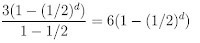## Saturday, 27 February 2010

### A Serendipitous Coincidence? The First-Ever Pursuit Problem.

Just reading through some old copies of the Mathematical Spectrum from my great source of mathematical periodicals, Dave Renfro. Intrigued by a couple of posts about a problem from the ancient Chinese Chiu Chang Suan Shu or Jiuzhang suanshu (Nine Chapters of Mathematical Art...about 150 BC) submitted by David Singmaster.

The problem: "A water weed grows 3 feet on the first day, and its growth on each succeeding day is half that on the preceding day. A reed grows 1 foot on the first day and its growth on each successive day is twice that of the preceding day? When are they of equal size?"

Go ahead, stop reading for a minute and try to solve it because I give an answer (actually two different ones) below and I don't want to spoil the fun.

The interesting thing to me, was the two letters of solution. My pre-calc students came through exponential growth and decay a few chapters ago, so they would approve of the first solution that was submitted. It suggested that we assume that the height of the water weed was growing according to the exponential function hw= 3 (1.5)d-1. The reed would reach a height of hr=3d-1. Setting these equal we would find the heights are equal when d= log26; or at about 2.585 days. They also pointed out that the mutual heights would be 5.705 feet.

Simple, quick, and "Wrong" according to the next commenter. They pointed out that a careful reading of the problem stated that the water weed "growth on any succeeding day is half that of the preceding day", would meant that it grew 3 feet the first day, and then successively it would grow 3/2, 3/4, 3/8 ... feet on each day.. to find the height we should sum this geometric series. So he suggests the height of the water weed would be hw=. This would result in the weed being somewhat shorter after each day than in the previous solution. In the same way, the reed should, on successive days, change by 1, 2, 4, 8 etc feet, so it would have a height of 2d-1 after d days. Setting these equal we see that the two plants will both reach a height of five feet (exactly) after d= log26; or at about 2.585 days.???? WAIT, that sounds familiar....where have I ... Oh YEAH!!!, that was the answer to the problem done the first way? WOW, what a lucky coincidence........ Well, NO... the good Professor who posed the problem stepped up to assure us that, in fact, if you used those same two approaches to similar problems they would always reach equal heights at the same time... (can you prove that???)

Try for yourself. I assumed similar means that the shorter grows at r times its previous days amount, and the taller at 1/r times the previous day. Try a few. In fact, it is frequently the case that the second method gives an integer solution, and when it is not, it seems pretty easy to adjust the growth on the first day of the two plants to make it come out an integer. If we call the first day growths W and R, then the solution works out to logr(rb/a); where r is the common ratio of the plant whose growth each day is increasing.

Dr Singmaster points out that this ancient text solves both quadratics and cubics, as well as systems of equations (including indeterminate systems with more unknowns than equations) using the "modern" method of elimination. It also is the earliest known text to have used negative numbers, and includes the rules for all the arithmetic operations. It is also the oldest know source of chase or pursuit problems, such as this one. This is problem 11 of the 7th chapter. I had the good fortune to sit with my beautiful Jeannie as the only two non-Chinese speakers at a discussion about the text at the Needham Research Institute in Cambridge by a group of English and Chinese experts. The amount I was able to take in is a credit to the patience of the gracious hosts.

Sue VanHattum said...

I don't understand the first method.

1.5^(d-1) is exponential growth, but the water weed has growth that's expressed by exponential decay. I also don't get why the reed would be 3^(d-1)??

I did it like the 2nd method. Cool problem.

Pat's Blog said...

Well, I'm explaining someone else's reasons, but I assume they reasoned that if the first day was 3 and the next day was half as much that was a fifty percent growth rate... so the exponential model would be Start(1+.50)^n... and he did this on both plants.
The actual time by the Chinese solution was the same as both of these, but they assumed the plant grew linearly during each day.. so they got even a different height..(I know, I have Dr. Singmasters word on it).

Pat's Blog said...

Sue,

Wow, that seemed more terse than I meant it to be..if that was not clear, please give me a second chance to explain.